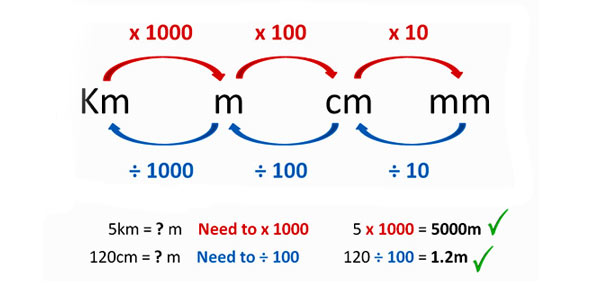# Heat, Energy Conversion, And Heat Transfer

6 Questions | Total Attempts: 417SettingsOnly select one answer unless otherwise specified. Please take your time. If you have any questions raise you hand and I will answer without giving the answer away. Good luck to all who may take this quiz.

Related Topics
• 1.
Why are metals generally more conductive than other materials?
• A.

Because of their electrons

• B.

Because of their protons

• C.

Because of their neutrons

• D.

None of the above

• 2.
True or False: Energy in the universe is never lost.
• A.

True

• B.

False

• 3.
How is energy "lost" when you drop a bouncy ball from 1 foot in the air and it only bounces 1/2 a foot from the ground?
• A.

Heat is created when the ball bounces

• B.

Sound is created when the ball bounces

• C.

Friction

• D.

All of the above

• 4.
What is work?
• A.

A paying occupation

• B.

Thinking

• C.

Force multiplied over distance

• D.

Energy

• 5.
What are the two main types of energy?
• A.

Kinetic and mechanical

• B.

Potential and Thermal

• C.

• D.

Kinetic and potential

• 6.
What is energy measured in?
• A.

Joules

• B.

Degrees

• C.

Inches

• D.

Miles